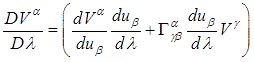## Pages

### 11. Parallel Transport and Geodesic

We now describe briefly about the concept of parallel transport and the geodesics.
Let us imagine a curve parameterized by $\lambda$

Consider the change of a vector $\vec{V}$ along the curve; we then have the following,This is a vector with components and we represent as follows,$(1)$

This is variously known as intrinsic, absolute or the total derivative. Note that we have made use of the chain rule in the above expression.

Then we can now come to a generalization of the concept of parallel transport. Imagine a vector $\vec{V}$ makes a differential displacement along the curve. We then have equation$(1)$. Now if the vector is displaced by an infinitesimal amount we can say that vectors remain parallel. Or in other words “If a vector $\vec{V}$ is “parallel transported” along a line then,

GEODESIC:
The concept of parallel transport can be used to extend the idea of “straight” lines to curved spaces.

We say that a curve is a straight line in a particular coordinate system if a vector parallel transports its own tangent vector. In other words, straight lines in curved spaces are defined by setting, $V^{\alpha }=du_{\beta }/{dx}$.
We then have as follows,

This is then equation of the geodesics. It is interesting to note here that the equation of the geodesics is of the second order differential equation, similar to those of that of the Newton’s force law or the equation of the motion. It won’t be surprising to find that this is in fact the general equation of motion in curved spacetime.